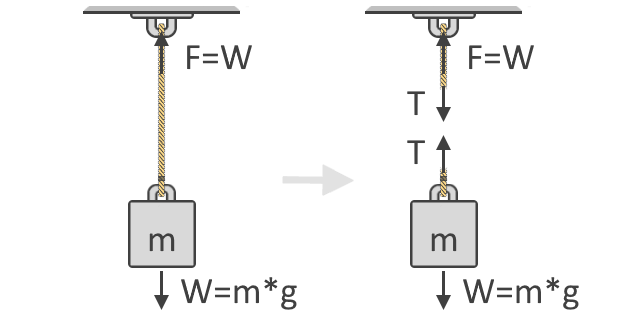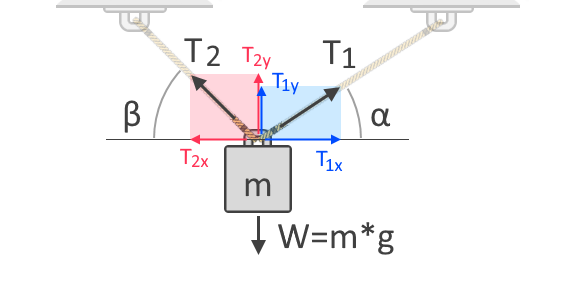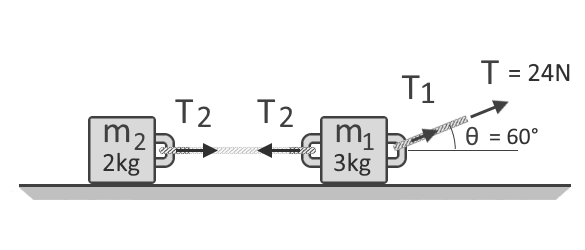# Tension Calculator

Created by Kenneth Alambra
Reviewed by Bogna Szyk and Jack Bowater
Last updated: Jun 20, 2022

This tension calculator will teach you how to find the tension force in a rope or string used to lift an object. In this article, you can also learn how to find tension in ropes used to pull an object on a frictionless surface. You will also see in this rope or string tension calculator a variety of free-body diagrams to further understand how to calculate tension forces. If you want to learn more about tension and the tension force formula, read on!

## What is tension force?

Imagine that you pick up a basketball from the ground. You would feel the ball's weight in your hands because of the force of gravity acting on the ball's mass. Now imagine tying a rope around the ball, which you then use to lift the ball again. You would still feel the ball's weight through the rope. In this situation, the rope is now in tension. What keeps the rope together is called the tension force. Cutting the rope will release the tension force and put the ball in free fall.

Tension force is an axial force that passes through an object that pulls, like a rope, string, or chain. We can also observe tension force in other materials, like rods and bars, given that they are subjected to external pulling or tensile loads. Materials with high tensile strength make the best rods and bars as they don't break easily when subjected to tension forces. You can check our stress-strain calculator, which discusses elasticity, to learn more about tensile strength.

Tension force is also a great example of Newton's Third Law of Motion. Newton's Third Law of Motion states that when a body exerts a force on a second body, the second body exerts an equal force in the opposite direction back onto the original body. Tension force is a reactive force that counteracts an external pulling force. This characteristic of tension force is the reason why it is, in a way, quite similar to the normal force, which you can learn more about by visiting our normal force calculator.

## Newton's Second Law of Motion

To calculate the tension that acts in a rope, we first need to understand Newton's Second Law of Motion. Newton's Second Law of Motion states that the sum of the forces acting on an object of constant mass is equal to the mass of that object multiplied by its acceleration. We can also express this statement as an equation:

ΣF = m * a

where

• Σ (sigma) denotes the summation of forces F;
• m is the mass of the object; and
• a is the acceleration.

For an object suspended by a rope, we can use gravitational acceleration, g, as its acceleration. Gravitational acceleration gives us the value of its weight in terms of force, like in Newtons or pound-force. If the object is moving at a different acceleration, we should use its actual acceleration for the calculation. However, this tension calculator only determines the tension forces in cases of static equilibrium.

This statement means that this tool only considers objects at rest in a given system. In this tension calculator, we also assume that ropes are massless and, therefore, do not contribute anything to the tension forces. We also assume that the masses or objects are in a vacuum and do not experience friction or air resistance towards their surroundings.

## How to calculate tension in ropes suspending an object

We can see in the illustration below that the force, F, needed to lift the object is equal to the weight, W, of the object. This idea is the fundamental concept that underlies our tension force formula. Also shown below is the free-body diagram of the object which shows the tension forces, T, acting in the string. As you can see, the tension forces come in pairs and in opposite directions:Following Newton's Second Law of Motion, we can then express the summation of forces using the free-body diagram of the object, as shown on the right side of the illustration above. We use free-body diagrams to show the different directions and magnitudes of the forces that act on a body. In equilibrium, these forces should all equate to zero. Considering all upward forces as positive and downwards as negative, our equation is:

ΣF↑ = 0 = T + (-W)

T = W

where weight, W, becomes negative since it is directed downwards. By transposing W to the other side of the equation, we can now see that the tension force in the rope is equal to the weight of the object it carries, as also shown above.

If we use more ropes to lift the object, the total tension force gets divided up into the ropes. The tension force in each rope depends on their angles with respect to the direction of the force it opposes. To further understand this, let us consider another free-body diagram of an object suspended by two ropes, as shown below:In the free-body diagram shown above, we can see the horizontal and vertical components of the tension forces, T₁, and T₂. Forces are vectors, which means they always have both magnitudes and directions. Like all vectors, forces can be expressed in these components which gives the force's influence along the horizontal and vertical axes. T₁ₓ and T₂ₓ are the vertical components of T₁ and T₂, respectively. On the other hand, T₁ᵧ and T₂ᵧ are the vertical components of the same forces, respectively. Since gravity acts on the object in the vertical axis, we need to consider the tension forces' vertical components for our summation of forces as follows:

ΣF↑ = 0 = T₁ᵧ + T₂ᵧ + (-W)

W = T₁ᵧ + T₂ᵧ

Because we also know the angles of the tension forces, we can express T₁ᵧ and T₂ᵧ in terms of T₁ and T₂, respectively, with the help of trigonometric functions:

T₁ᵧ = T₁ * sin(α)

T₂ᵧ = T₂ * sin(β)

W = T₁ * sin(α) + T₂ * sin(β)

We can also say that for the system to be in equilibrium, the object should not move horizontally or along the x-axis. Therefore, the horizontal components of T₁ and T₂ must then equate to zero. Also, with the help of trigonometry, we can express T₁ₓ and T₂ₓ in terms of T₁ and T₂, respectively:

T₁ₓ = T₂ₓ

T₁ * cos(α) = T₂ * cos(β)

If we divide both sides by cos(α), we obtain an equation wherein T₁ is expressed in terms of T₂ and the angles:

T₁ = T₂ * cos(β) / cos(α)

We can then use this equation to solve for T₂ by substituting T₂ * cos(β) / cos(α) as the T₁ in our summation of forces equation, as shown below:

W = T₁ * sin(α) + T₂ * sin(β)

W = T₂ * [cos(β) / cos(α)] * sin(α) + T₂ * sin(β)

W = T₂ * [cos(β) * sin(α) / cos(α) + sin(β)]

T₂ = W / [cos(β) * sin(α) / cos(α) + sin(β)]

Finally, if we multiply this entire equation by cos(β) / cos(α) as we derived in the value of T₁ in terms of T₂, and then simplifying everything, we get this equation:

T₁ = W / [cos(β) * sin(α) / cos(α) + sin(β)] * [cos(β) / cos(α)]

T₁ = W / [cos(β) * sin(α) / cos(α) + sin(β)] * [cos(β) / cos(α)]

T₁ = W / [cos(α) * sin(β) / cos(β) + sin(α)]

Now all you need to know are the angles of the tension ropes with respect to the horizontal. If an angle from the vertical is given, just subtract this angle from 90°. Doing so will provide you with the angle from the horizontal. However, if you are given other values of angles that may be greater than 90° or even 180°, you might want to check out our reference angle calculator to help you determine the angle you need. After determining the values for the variables in our tension force formulas, we can now solve for the tension forces.

## How to find tension in ropes while pulling an object

How to find the tension force on an object being pulled is just like when the object is hung. The only difference is that we first need to compute the acceleration of the entire system and sum all of the of forces along the horizontal. If the rope is at an angle from the level of the floor, we need to compute for the horizontal component of the pulling force too.

Let us take a look at the example below to better understand how to find the tension force in a rope pulling one or two objects. In this example, two objects are being pulled by a single applied pulling force. Another rope is pulling the second object, which is attached to the first object, as shown below:This illustration shows that the masses of m₁ and m₂ are 3 kg and 2 kg, respectively. The sum of these two masses gives the total mass of the system, 5 kg. We also need to determine the horizontal component of the pulling force, T = 24 N, that is at an angle θ = 60°. If we use the trigonometric functions again, we can say that the horizontal component of the pulling force is equal to 24 N * cos(60°), which is equal to 12 N. Now that we know the pulling force's horizontal component and the total mass of the system, we can now calculate the acceleration, a, of the system as follows:

F = m * a → a = F / m

a = 12 N / 5 kg = 2.4 m/s²

After we have found the acceleration of the system, we can use Newton's Second Law of Motion again to calculate the system's rope or string tension. To do this, multiply the acceleration by the mass that the rope is pulling. For T₂, its free-body diagram shows us it is only responsible for the mass of m₂, we can say that T₂ = a * m₂. With that said, T₂ = (2.4 m/s²) * (2 kg) = 4.8 N. On the other hand, T₁ is the tension force that pulls both the weight of m₁ and m₂. However, we already have the value for T₁, which is simply equal to T = 24.0 N. Therefore, T₁ = 24.0 N.

In our example, if the left and right ropes were just a single rope, we could compare this setup to a pulley system. A pulley is a simple machine that takes advantage of tension forces in ropes to gain mechanical advantage. You can check out our pulley calculator and belt length calculator (which is a two-pulley system) to learn more about mechanical advantage and tension.

Now that you know how to find tension in a rope, perhaps you would also like to try our weight on other planets calculator. There you can determine the weight of an object if it was on another planet. You could then input that weight into our tension calculator to solve for tension forces if you were on another planet. On the other hand, if you're into music, you might want to explore the relationship of string tension and sound frequency in stringed instruments through our fret calculator.

## FAQ

### How do I calculate the tension of a rope at an angle?

To calculate the tension of a rope at an angle:

1. Find the angle from the horizontal the rope is set at.
2. Find the horizontal component of the tension force by multiplying the applied force by the cosine of the angle.
3. Work out the vertical component of the tension force by multiplying the applied force by the sin of the angle.
4. Add these two forces together to find the total magnitude of the applied force.
5. Account for any other applied forces, for example, another rope, gravity, or friction, and solve the force equation normally.

### How do I calculate the tension produced by a 10kg box on two ropes suspended at 60 degrees?

To determine the tension in this case:

1. Determine the vertical and horizontal components of the tensile forces on the ropes:
• T₁ = T₁ sin(60) + T₁ cos(60)
• T₂ = T₂ sin(60) + T₂ cos(60)
2. The vertical (sine) components balance the weight:
• T₁ sin(60) + T₂ sin(60) = √3 (T₁ + T₂)/2 = 98 N
3. The tension force's horizontal (cosine) components balance each other:
• T₁ cos(60) = T₂ cos(60) or T₁ = T₂
4. Solve the equations:
• 98 N = √3 T₁ or, T₁ = T₂ = 56.58 N

### How do I find tension in two ropes at the same angle of suspension?

When the angles of suspension (α) are the same for two ropes, the magnitude of tension (T) becomes the same for both ropes. To determine the magnitude of tension use the equation 2T sin(α) = m × g where m × g represents is the weight of the suspended object.

### Is tension a contact force?

Yes, tension is a contact force. Tension arises in a rope or a cable upon it being stretched and tensile forces are transmitted through the rope from one end to the other.

Kenneth Alambra
Tension scenario
Object is hanging
Number of ropes
1Input values
Object's mass, m
lb
Weight, W
lbf
Output value
Tension in the rope, T
lbf
People also viewed…

### Black Hole Collision Calculator

The Black Hole Collision Calculator lets you see the effects of a black hole collision, as well as revealing some of the mysteries of black holes, come on in and enjoy!

### Chilled drink

With the chilled drink calculator you can quickly check how long you need to keep your drink in the fridge or another cold place to have it at its optimal temperature. You can follow how the temperature changes with time with our interactive graph.

### Rotational kinetic energy

Rotational kinetic energy calculator finds the energy of an object in rotary motion.

### Water heating

Use the water heating calculator to determine how much time and energy you'll need to increase the temperature of the water.